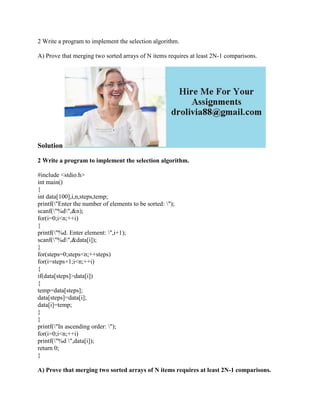Diese Präsentation wurde erfolgreich gemeldet.

# 2 Write a program to implement the selection algorithm- A) Prove that.docx

Anzeige
Anzeige
Anzeige
Anzeige
Anzeige
Anzeige
Anzeige
Anzeige
Anzeige
Anzeige
Anzeige×

1 von 2 Anzeige

# 2 Write a program to implement the selection algorithm- A) Prove that.docx

2 Write a program to implement the selection algorithm.
A) Prove that merging two sorted arrays of N items requires at least 2N-1 comparisons.
Solution
2 Write a program to implement the selection algorithm.
#include <stdio.h>
int main()
{
int data,i,n,steps,temp;
printf(\"Enter the number of elements to be sorted: \");
scanf(\"%d\",&n);
for(i=0;i<n;++i)
{
printf(\"%d. Enter element: \",i+1);
scanf(\"%d\",&data[i]);
}
for(steps=0;steps<n;++steps)
for(i=steps+1;i<n;++i)
{
if(data[steps]>data[i])
{
temp=data[steps];
data[steps]=data[i];
data[i]=temp;
}
}
printf(\"In ascending order: \");
for(i=0;i<n;++i)
printf(\"%d \",data[i]);
return 0;
}
A) Prove that merging two sorted arrays of N items requires at least 2N-1 comparisons.
The observation about mergesort is that no matter suppose input array looks like, mergesort will divide
the array recursively into the same two sorted array. The way the input influences the way the algorithm works
in is the merge phase of the algorithm. A merge of two N-element sorted arrays requires at least N comparisons (this happens if one array\'s elements are all less than the other array\'s smallest element) and at most 2N-1 comparisons. In any case, merge requires 2N assignments.
So, merge requires Theta(N) time regardless of the input.
This implies that mergesort takes when marging two sorted arrays of N item requires at least 2N-1 comparisons.
.

2 Write a program to implement the selection algorithm.
A) Prove that merging two sorted arrays of N items requires at least 2N-1 comparisons.
Solution
2 Write a program to implement the selection algorithm.
#include <stdio.h>
int main()
{
int data,i,n,steps,temp;
printf(\"Enter the number of elements to be sorted: \");
scanf(\"%d\",&n);
for(i=0;i<n;++i)
{
printf(\"%d. Enter element: \",i+1);
scanf(\"%d\",&data[i]);
}
for(steps=0;steps<n;++steps)
for(i=steps+1;i<n;++i)
{
if(data[steps]>data[i])
{
temp=data[steps];
data[steps]=data[i];
data[i]=temp;
}
}
printf(\"In ascending order: \");
for(i=0;i<n;++i)
printf(\"%d \",data[i]);
return 0;
}
A) Prove that merging two sorted arrays of N items requires at least 2N-1 comparisons.
The observation about mergesort is that no matter suppose input array looks like, mergesort will divide
the array recursively into the same two sorted array. The way the input influences the way the algorithm works
in is the merge phase of the algorithm. A merge of two N-element sorted arrays requires at least N comparisons (this happens if one array\'s elements are all less than the other array\'s smallest element) and at most 2N-1 comparisons. In any case, merge requires 2N assignments.
So, merge requires Theta(N) time regardless of the input.
This implies that mergesort takes when marging two sorted arrays of N item requires at least 2N-1 comparisons.
.

Anzeige
Anzeige

### 2 Write a program to implement the selection algorithm- A) Prove that.docx

1. 1. 2 Write a program to implement the selection algorithm. A) Prove that merging two sorted arrays of N items requires at least 2N-1 comparisons. Solution 2 Write a program to implement the selection algorithm. #include <stdio.h> int main() { int data,i,n,steps,temp; printf("Enter the number of elements to be sorted: "); scanf("%d",&n); for(i=0;i<n;++i) { printf("%d. Enter element: ",i+1); scanf("%d",&data[i]); } for(steps=0;steps<n;++steps) for(i=steps+1;i<n;++i) { if(data[steps]>data[i]) { temp=data[steps]; data[steps]=data[i]; data[i]=temp; } } printf("In ascending order: "); for(i=0;i<n;++i) printf("%d ",data[i]); return 0; } A) Prove that merging two sorted arrays of N items requires at least 2N-1 comparisons.
2. 2. The observation about mergesort is that no matter suppose input array looks like, mergesort will divide the array recursively into the same two sorted array. The way the input influences the way the algorithm works in is the merge phase of the algorithm. A merge of two N-element sorted arrays requires at least N comparisons (this happens if one array's elements are all less than the other array's smallest element) and at most 2N-1 comparisons. In any case, merge requires 2N assignments. So, merge requires Theta(N) time regardless of the input. This implies that mergesort takes when marging two sorted arrays of N item requires at least 2N- 1 comparisons.